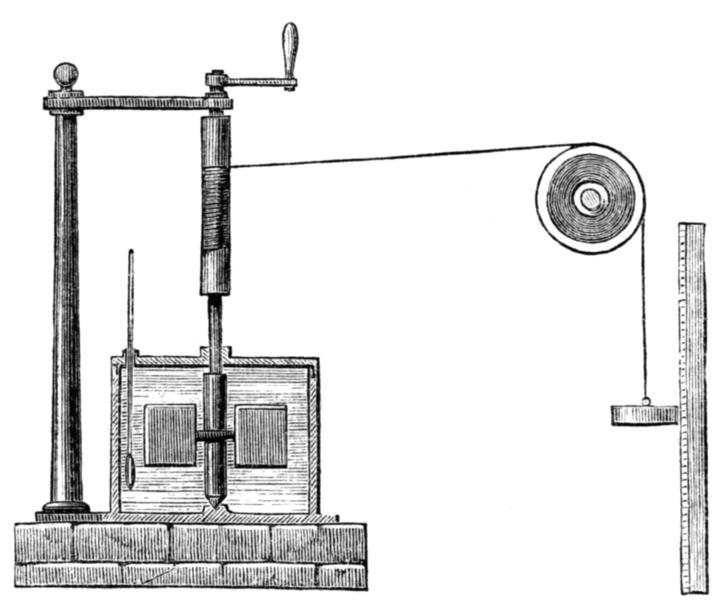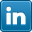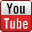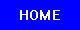﻿ James Prescott Joule: The Discovery of the Mechanical Equivalent of Heat

James Prescott Joule
The Discovery of the Mechanical Equivalent of Heat
Build a Joule Apparatus and Calculate the Mechanical Equivalent of Heat

At the end of the 18th century the prevailing heat theory was the, obsolete now, caloric theory, according to which heat consists of a fluid called caloric that flows from hotter to colder bodies. Caloric was also thought of as a weightless gas that could pass in and out of pores in solids and liquids.

### Count Rumford's Contribution

Benjamin Thompson, Count Rumford (1753 – 1814) was an Anglo-American physicist and inventor whose challenges to established physical theory were part of the 19th century revolution in thermodynamics.

While holding a position for the Bavarian army, Rumford’s experiments on gunnery and explosives led him to an interest in heat. Rumford had observed the large frictional amounts of heat generated by boring brass cannon barrels at the arsenal in Munich. Rumford immersed a cannon barrel in water and arranged for a specially blunted boring tool in order to generate more heat than usual. He showed that the water could be boiled within roughly two and a half hours and that the supply of frictional heat was seemingly inexhaustible since as long as the boring process takes place heat is generated.

Rumford also confirmed that no physical change had taken place in the material of the cannon by comparing the specific heats of the material machined away and that remaining.

Those findings, of Rumford, were incompatible with the caloric theory, speaking about liquids and gases passing between materials since anything that could be generated without limitation could not be a material substance such as a caloric fluid or gas. He contended that the only thing that boiled the water, in his cannon experiments, was the motion of the bore inside the barrel and its resulting friction.

Rumford published his findings in 1798 in his An Experimental Enquiry Concerning the Source of the Heat which is Excited by Friction, arguing that in his experiments heat was not the “caloric” but a form of motion.

Count Rumford suspected that there is an equivalence between heat and mechanical work with a definite conversion factor between the two.

However, Rumford made no attempt to further quantify the heat generated or to measure or calculate the mechanical equivalent of heat.

Though Rumford’s work met with a hostile reception, it was subsequently important in establishing the laws of conservation of energy later in the 19th century.

### Joule's Contribution

James Prescott Joule (1818 – 1889), an English physicist, calculated in 1843, a few decades after Rumford’s cannon experiments, the mechanical equivalent of heat in a series of experiments. In the most famous apparatus he built for this end, now called the Joule apparatus (see image below), a descending weight attached to a string caused a paddle immersed in water to rotate and heat the water. Joule supposed that the gravitational potential energy lost by the weight in descending was equal to the thermal energy (heat) gained by the water by friction with the paddle.

In this experiment, the friction and agitation by the paddle-wheel of the body of water, trapped in an insulated barrel, caused heat to be generated which, in turn, increased the temperature of the water. The temperature change ∆T of the water and the height of the fall ∆h of the weight m*g were recorded. Using these values, Joule was able to determine the mechanical equivalent of heat.

Specifically, Joule had experimented on the amount of mechanical work generated by friction needed to raise the temperature of a pound of water by one degree Fahrenheit and found a consistent value of 772.24 foot pound force (in English units) or 4.1550 J/cal (SI metric units) in comparison to the 4.1868 J/cal modern value – meaning that around 4.2 J were needed to raise the temperature of 1g of water by 1°.C - and that’s the mechanical equivalent of heat in its respective units (The Joule unit was introduced after Joule's times (after him) and he calculated the mechanical equivalent of heat in English units).

Joule contended that motion and heat were mutually interchangeable and that, in every case, a given amount of work (motion) would generate the same amount of heat. Moreover, he also claimed that heat was only one of many forms of energy (electrical, mechanical, chemical) and only the sum of all forms was conserved. Otherwise the calculated mechanical equivalent of heat is meaningless.

In 1845, James Joule reported his experiment in a paper On the mechanical equivalent of heat for the British Association meeting in Cambridge. http://www.chemteam.info...

### Topics of Interest

Others, like Julius von Mayer and Ludwig A. Colding, also contributed independently to the measuring and calculation of the mechanical equivalent of heat in the same time as Joule and also reached similar results, but at the end the credit went to Joule maybe because of simplicity and clarity of his experiments and ideas concerning the matter.

The concept of equivalence stated that motion and heat (and other types of energy) are mutually interchangeable and that in every case, a given amount of work would generate the same amount of heat, provided the work done is totally converted to heat energy. Those early energy pioneers like Joule, Mayer, Colding and others paved the way for the conservation of energy principle, definitively stated by Hermann von Helmholtz in 1847, and the establishment of the science of thermodynamics in the 19th century.

### Build a Joule Apparatus and Repeat Joule's ExperimentJoule's apparatus for measuring the mechanical equivalent of heat. A descending weight attached to a string causes a paddle immersed in water to rotate and the "work" of the falling weight is converted into "heat" by agitating the water and raising its temperature.

Before we begin our experiment, a little bit about the physical units involved. The following explanation refers to the more modern and popular SI system of units that can be converted with no much effort (also with the help of online converters) to English units if needed.

The small calorie or gram calorie (symbol: cal) is the energy needed to increase the temperature of 1 gram of water by 1 °C. This is about 4.2 joules.

The large calorie, kilogram calorie or food calorie (symbol: Cal) is the energy needed to increase the temperature of 1 kilogram of water by 1 °C. This is exactly 1000 small calories or about 4.2 kilojoules (4200 J).

In an attempt to avoid confusion, the large calorie is sometimes written as Calorie (with a capital C). This convention, however, is not always followed. Whether the large or small calorie is intended often must be inferred from context. When used in scientific contexts, the term calorie refers to the gram calorie (small calorie). In nutritional contexts, however, a larger unit is more useful. In such contexts the term calorie can be taken to refer to the kilogram calorie (symbol: kcal).

1 Joule is the energy consumed in applying a force of one newton through a distance of one metre (1 newton•metre or N•m).

Specific heat of water = the energy needed to increase the temperature of 1g of water by 1° C (by definition of the calorie) = 1 cal or 4.18 J (inferred from the mechanical equivalent of heat value). Take in account that other materials and liquids have different values for their specific heat.

Joule made a series of measurements and found that, on average a weight of 772 pounds falling through a distance of one foot would raise the temperature of one pound of water by 1° F. 772 pounds means around 350 kg – not so practical of a weight to experiment with. Therefore, Joule used a lesser weight falling over a greater distance, repeated a number of times.

In order to increase the temperature of 1kg (1 liter) of water by 1° C you’ll need some 4200 J of energy generated by the falling body. According to the potential energy loss of the falling body 4200=m*g*h where g the gravitational constant is around 10 m/s2 so our equation comes to 4200=10m*h or 420=m*h. If we are going to use a mass of 1kg and drop it from a height of 1 m, then we’ll need to repeat this procedure 420 times in order to conclude the experiment.

From this simple equation (420=m*h) is clear that increasing the mass m or the height h or using a sensitive thermometer say with digital display of 0.1°C, with no big effort we can reduce the repetitions needed to much less than one hundred.

We should take in consideration that some energy will be lost by the friction of the falling weight with the air, the string / rope friction, and heat transferred to the insulated barrel. So, your results will be a little higher than the 4.18 J/c. Therefore, the barrel must be caped and insulated as much as possible and the thermometer inserted through the cape not allowing contact with room air. And the experiment should be performed around 25 °C room temperature since physical values are defined for room temperature.

In order to achieve accurate results as much as possible it is recommended to repeat the entire experiment a few times, remove the extremes and calculate the average.

Another option for experimentation with Joule's apparatus is to try to measure the specific heat of other liquids than water.

### Books